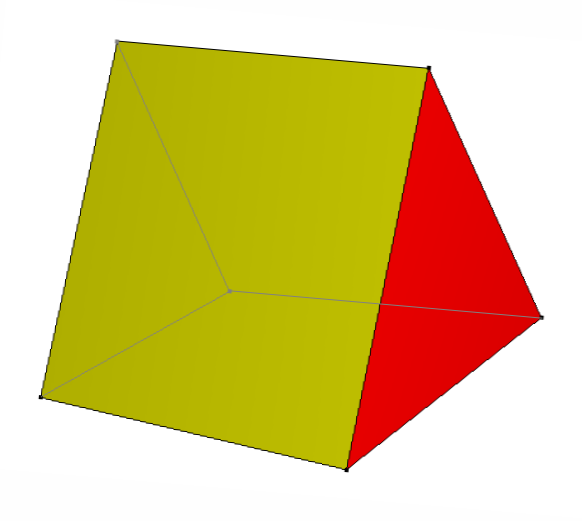# Difference between Triangular Prism and Triangular Pyramid Tetrahedron

Both Triangular prism and a triangular pyramid tetrahedron are polyhedrons. A polyhedron, in geometry is a three-dimensional solid with straight edges and flat faces. Since both prism and pyramid are polyhedrons, most people believe they are the same, while they are not. A pyramid is formed by joining a polygonal base with a point called apex in such a way that each edge of the base forms a triangle. The base of a pyramid can be any polygon shape, such as trilateral, quadrilateral etc.

The triangular prism has a triangular base and rectangular sides, whereas a tetrahedron has a polygonal base and triangular sides. A triangular prism has five sides, six vertices and nine edges. A triangular pyramid, on the other hand, has four sides, four vertices and six edges.

### Instructions

• 1

In case of a triangular prism, the area of cross section along the axis perpendicular to the base does not change. However, the cross sectional area in tetrahedrons changes along the axis through the base (decreases with increase in distance from the base). If both triangular prisms and triangular pyramids have the same triangle as the base and the same height, the volume of the former will be three times the volume of the latter.

Triangular prism

A triangular prism is a solid geometric shape with triangular base, i.e. the cross sections of the prism parallel to the base are triangles. In geometry, a triangular prism is a pentahedron with opposite sides parallel to each other, while the side normal to the remaining three sides lies in the same plane. The bases of a prism are always triangles, while the other sides are rectangular in shape.

A right prism is the one in which the planes of the bases make an angle of exactly 90 degrees with the other sides. Volume of a triangular prism is calculated by multiplying the area of the base triangle with the length between the two bases, also called the height of the prism.

Volume = base area × height

Image courtesy: commons.wikimedia.org• 2

Triangular Pyramid Tetrahedron

A triangular pyramid is a solid geometric shape consisting of a polygonal base and triangular faces. It is the simplest form of pyramids and is also called a tetrahedron. The four triangular sides of a tetrahedron can be different, however if all the four triangles are equilateral, it is called a regular tetrahedron.

The volume of a tetrahedron can be calculated using the formula:

Volume = (1/3) base area × height

Where height refers to the distance between the base and the tip or apex of the tetrahedron.

Image courtesy: k6-geometric-shapes.com•